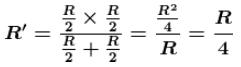Courses

# Current Electricity MCQ Level - 1

## 10 Questions MCQ Test Basic Physics for IIT JAM | Current Electricity MCQ Level - 1

Description
This mock test of Current Electricity MCQ Level - 1 for Physics helps you for every Physics entrance exam. This contains 10 Multiple Choice Questions for Physics Current Electricity MCQ Level - 1 (mcq) to study with solutions a complete question bank. The solved questions answers in this Current Electricity MCQ Level - 1 quiz give you a good mix of easy questions and tough questions. Physics students definitely take this Current Electricity MCQ Level - 1 exercise for a better result in the exam. You can find other Current Electricity MCQ Level - 1 extra questions, long questions & short questions for Physics on EduRev as well by searching above.
QUESTION: 1

### A wire is stretched to n times its length. Then the resistance now will increase by :

Solution:

M = constant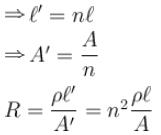The correct answer is: n2 times

QUESTION: 2

### A cell has an emf 1.5 V when connected across in an external resistance of 2Ω the terminal potential difference falls to 1.0 V. The internal resistance of the cell is :

Solution:

Internal resistance of the cell is given by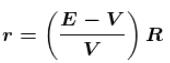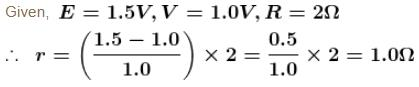QUESTION: 3

### n equal cell having e.m.f. E and internal resistance  r, are connected in circuit of a resistance  R. Same current flows in circuit either they connected in series or parallel, if :

Solution:

Current when cells are connected in series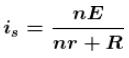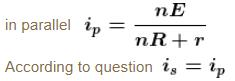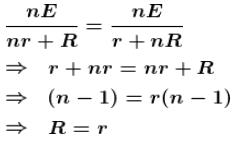The correct answer is: R = r

QUESTION: 4

The potential difference between point A  and B  is :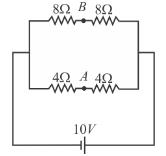Solution:
QUESTION: 5

A wire is bent in the form of a triangle now the equivalent resistance between its one end and the mid point of the side is :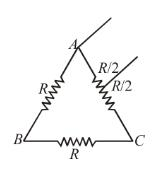Solution:

Resistance RR  and R/2 are in series. Their resultant resistance.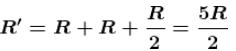Resistance R'  and R/2 are in series. Their resultant resistance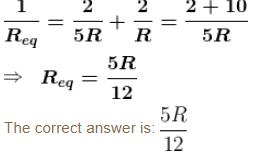QUESTION: 6

There is a voltmeter in a circuit. In order to triple its range, the resistance of how much value should be used?

Solution:

If R  is the resistance of the voltmeter and  current is flowing through it, then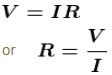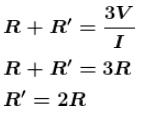QUESTION: 7

Nine resistors each of resistance R  are connected in the circuit as shown in figure. The net resistance between A  and B is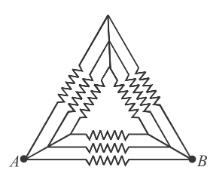Solution: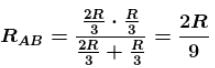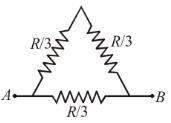QUESTION: 8

The resistance of an ammeter is 13Ω and its scale is graduated for a current upto 100 A. After an additional shunt has been connected to this ammeter it becomes possible to measure currents upto 750 A  by this meter. The value of shunt resistance is :

Solution:

The potential differences across ammeter and shunt is same. Let  ia is the current flowing through ammeter and i is the total current. So, a current  i - ia  will flow through. shunt resistance.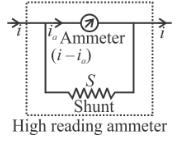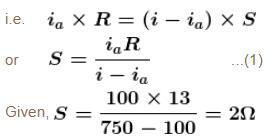QUESTION: 9

The value of i1/i2 for a given diagram is :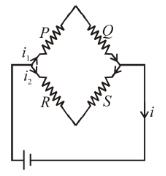Solution:
QUESTION: 10

When a wire of uniform cross-section a length ℓ and resistance R is bent into a complete circle, resistance between two of diametrically opposite points will be :

Solution:

When wire is bent to form a complete circle then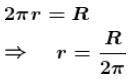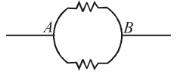Resistance of each semicircle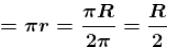Thus, net resistance is parallel combination of two semicircular resistances